Next: Quality Factor Up: One-Dimensional Motion Previous: Simple Harmonic Motion

Damped Oscillatory Motion

According to Equation (78), a one-dimensional conservative system which is slightly perturbed from a stable equilibrium point (and then left alone) oscillates about this point with a fixed frequency and a constant amplitude. In other words, the oscillations never die away. This is not very realistic, since we know that, in practice, if we slightly perturb a dynamical system (such as a pendulum) from a stable equilibrium point then it will indeed oscillate about this point, but these oscillations will eventually die away due to frictional effects, which are present in virtually all real dynamical systems. In order to model this process, we need to include some sort of frictional drag force in our perturbed equation of motion, (77).

The most common model for a frictional drag force is one which is always directed in the opposite direction to the instantaneous velocity of the object upon which it acts, and is directly proportional to the magnitude of this velocity. Let us adopt this model. So, our drag force can be written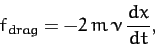(82)

where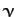is a positive constant with the dimensions of frequency. Including such a force in our perturbed equation of motion, (77), we obtain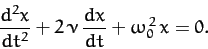(83)

Thus, the positive constantparameterizes the strength of the frictional damping in our dynamical system.

Equation (83) is a linear second-order ordinary differential equation, which we suspect possesses oscillatory solutions. There is a standard trick for solving such an equation. We search for complex solutions of the form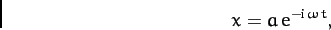(84)

where the constants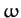and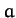are both, in general, complex. Of course, the physical solution is the real part of the above expression: i.e.,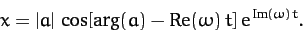(85)

Clearly, the modulus and argument of the complex amplitude,, determine the amplitude (at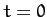) and phase of the oscillation, respectively, whereas the real and imaginary parts of the complex frequency,, determine its frequency and growth-rate, respectively. Note that this method of solution is only appropriate for linear differential equations. Incidentally, the method works because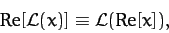(86)

whereis a complex variable, and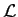some real linear differential operator which acts on this variable. [A linear operator satisfies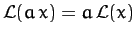for alland, whereis a constant. The differential operator appearing in Equation (83) is clearly of this type.]

Substituting Equation (84) into Equation (83), we obtain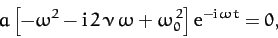(87)

which reduces to the following quadratic equation for[since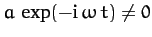]: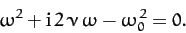(88)

The solution to this equation is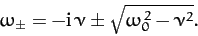(89)

Thus, the most general physical solution to Equation (83) is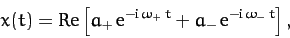(90)

where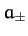are two arbitrary complex constants.

We can distinguish three different cases. In the first case,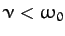, and the motion is said to be underdamped. The most general solution is written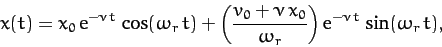(91)

where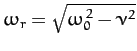,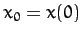, and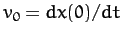. It can be seen that the solution oscillates at some real frequency,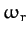, which is somewhat less than the natural frequency of oscillation of the undamped system,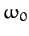, but also decays exponentially in time at a rate proportional to the damping coefficient,.

In the second case,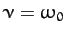, and the motion is said to be critically damped. The most general solution is written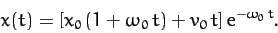(92)

It can be seen that the solution now decays without oscillating.

In the third case,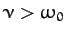, and the motion is said to be overdamped. The most general solution is written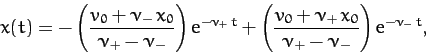(93)

where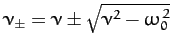. It can be seen that the solution again decays without oscillating, except there are now two independent decay rates. The largest,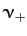, is always greater than the critically damped decay rate,, whereas the smaller,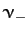, is always less than this decay rate. This means that, in general, the critically damped solution is more rapidly damped than either the underdamped or overdamped solutions.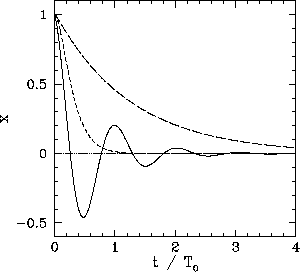Figure 5 shows typical examples of underdamped (i.e.,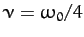), critically damped (i.e.,), and overdamped (i.e.,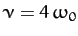) solutions, calculated with the initial conditions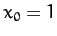and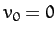. Here,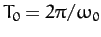. The three solutions correspond to the solid, short-dashed, and long-dashed curves, respectively.Next: Quality Factor Up: One-Dimensional Motion Previous: Simple Harmonic Motion
Richard Fitzpatrick 2011-03-31